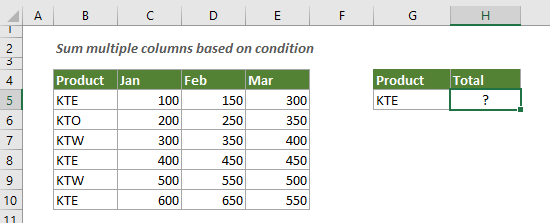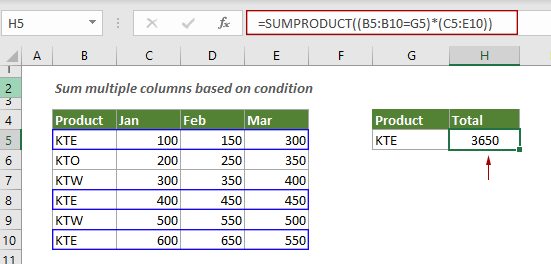## Sum multiple columns if one criterion is met in Excel

This tutorial will guide you how to sum values in multiple columns based on the same criterion in Excel.

#### How to sum multiple columns based on one criteria?

Look at the example below, suppose you have a list of products with three months of sales, and you want to sum all sales for the product “KTE”, you can follow the steps below.Generic Formulas

=SUMPRODUCT((criteria_rng=criteria)*(sum_range))

Arguments

Criteria_range: The range of cells that you want evaluated by the criterion;
Criteria: The criterion that defines which cells in the criteria_range will be summed;
Sum_range: The range of cells to sum.

How to use this formula?

Select a blank cell, copy or enter the formula below and press the Enter key to get the result.

=SUMPRODUCT((B5:B10=G5)*(C5:E10))Note:

1) You can directly type the criterion into the formula and enclose it in double-quotes;
=SUMPRODUCT((B5:B10="KTE")*(C5:E10))
2) You can use the SUM and IF functions together to do the same calculation. Don’t forget to activate this formula by pressing Ctrl + Shift + Enter keys.
=SUM(IF(B5:B10="KTE",C5:E10))

Explanation of the formula

=SUMPRODUCT((B5:B10=G5)*(C5:E10))

(B5:B10=G5): Here checks if cells in the range B5:B10 equal to “KTE” and returns an array like this {TRUE;FALSE;FALSE;TRUE;FALSE;TRUE};
{TRUE;FALSE;FALSE;TRUE;FALSE;TRUE}*(C5:E10): Each TRUE or FALSE in the array is multiplied by values in range C5:C10 (All TRUEs and FALSEs are converted to 1s and 0s), and a new array comes out as {100,150,300;0,0,0;0,0,0;400,450,450;0,0,0;600,650,550};
SUMPRODUCT({100,150,300;0,0,0;0,0,0;400,450,450;0,0,0;600,650,550}): The SUMPRODUCT function adds up all the numbers in the array and returns the final result as 3650.

#### Related functions

Excel SUMPRODUCT function
The Excel SUMPRODUCT function can be used to multiply two or more columns or arrays together, and then get the sum of products.

#### Related formulas

Sum the last N columns
To sum values in the last n columns, normally you may need to calculate the sum of each column and then add up the results to get the final sum. This tutorial provides a formula based on the INDEX function to help you easily get it done.

Sum top N values or top N values with criteria
This step-by-step tutorial demonstrates how to use the SUMPRODUCT function with the LARGE function to sum the top N values in a range and sum the top N values in a range with criteria in Excel.

Sum values based on column or both column and row
This tutorial provides different formulas based on the SUMPRODUCT function to help you sum values based on a column header, and sum values based on both column and row criteria.

Sum values in last N days based on criteria
To sum values in last N days based on specific criteria in Excel, you can apply a formula based on the SUMIF and TODAY function.

### The Best Office Productivity Tools

#### Kutools for Excel - Helps You To Stand Out From Crowd

 Popular Features: Find, Highlight or Identify Duplicates  |  Delete Blank Rows  |  Combine Columns or Cells without Losing Data  |  Round without Formula ... Super VLookup: Multiple Criteria  |  Multiple Value  |  Across Multi-Sheets  |  Fuzzy Lookup... Adv. Drop-down List: Easy Drop Down List  |  Dependent Drop Down List  |  Multi-select Drop Down List... Column Manager: Add a Specific Number of Columns  |  Move Columns  |  Toggle Visibility Status of Hidden Columns  |  Compare Columns to Select Same & Different Cells ... Featured Features: Grid Focus  |  Design View  |  Big Formula Bar  |  Workbook & Sheet Manager | Resource Library (Auto Text)  |  Date Picker  |  Combine Worksheets  |  Encrypt/Decrypt Cells  |  Send Emails by List  |  Super Filter  |  Special Filter (filter bold/italic/strikethrough...) ... Top 15 Toolsets:  12 Text Tools (Add Text, Remove Characters ...)  |  50+ Chart Types (Gantt Chart ...)  |  40+ Practical Formulas (Calculate age based on birthday ...)  |  19 Insertion Tools (Insert QR Code, Insert Picture from Path ...)  |  12 Conversion Tools (Numbers to Words, Currency Conversion ...)  |  7 Merge & Split Tools (Advanced Combine Rows, Split Excel Cells ...)  |  ... and more

Kutools for Excel Boasts Over 300 Features, Ensuring That What You Need is Just A Click Away...#### Office Tab - Enable Tabbed Reading and Editing in Microsoft Office (include Excel)

• One second to switch between dozens of open documents!
• Reduce hundreds of mouse clicks for you every day, say goodbye to mouse hand.
• Increases your productivity by 50% when viewing and editing multiple documents.
• Brings Efficient Tabs to Office (include Excel), Just Like Chrome, Edge and Firefox.